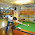### Probability Puzzle: Expected Number of Expression Evaluations

Source: Asked for a data scientist position at a technology startup in financial services sector in London

Problem:

Given python code to calculate maximum in an array of integers x

def find_max(x):

max_num = x

for i in x[1:]:
if i > max_num:
max_num = i << Expected number of times this expression was evaluated

return max_num

Calculate the expected number of times the expression max_num = i was evaluated, given that array x was taken from a uniform random distribution.

1.answer is 1/2 + 1/3 + 1/4...+ 1/n

probability that j-th element is the maximum till now = 1/j

sum over all such j's from 2 to n

1.2.Assuming total no of elements N. (N+1)/2

3.Assumption : Elements come in random order.
Consider the ith iteration, the probability that the ith element is the largest amongst the first i elements is simply 1/i since all are equally likely to be largest. So E[no. of times the line is evaluated for ith iteration] = 1/i = E_i. So,
E[total number of assignments to var max_num] = summation of E_i for i=1 to n. This is approximately LogN for large N.

4.n/2 by there are infinitely many integers on either side of any integer x and thus probability that next number in array is greater than current maximum is 1/2. Thus expected number is n/2

5.Since we are talking about an integer array, the uniform distribution from which the elements are picked is discrete in nature. And if the range of this distribution is finite, the statement "the probability that the ith element is the largest amongst the first i elements is simply 1/i since all are equally likely to be largest" is not true.

Consider, for example, a range consisting of only a single integer. Then the expected value is clearly 0. Or a range containing less than LogN integers, then the expected value is clearly less than LogN. The argument holds, however, if the probability of repitition of elements in the array is negligible, which is true if the range is infinite or the array contains real numbers instead of integers.

6.This comment has been removed by the author.